# Displacement, Velocity, and Acceleration

Measurement and Units
The units associated with numbers are important in physics. The units tie the numbers to real and measureable physical quantities. For example, distance can be measured in many different units, such as inches, centimeters, miles, kilometers, or light years. In physics, the international system of units, "SI", is used. SI uses metric measurements, but SI also defines a "base" set of units that is used to build "compound" units that are given their own names. The SI base set of units are the meter (m) for measuring distance, the kilogram (kg) for measuring mass, the second (s) for measuring time, the Ampere (A) for measuring electric current, the Kelvin (K) for measuring temperature, the mole (mol) for measuring the amount of a substance, and the candela (cd) for measuring the intensity of light.

Vectors in One Dimension
Many measurable values only have a number and a unit. These quantities, like mass or temperature, are called "scalar" values. Other measurable quantities have a value (also known as a "magnitude") and a direction. Values that relate to motion are an example. The direction that something travels is important. Telling someone, "drive one mile east" is very different than telling the person, "drive one mile south". Quantities that have a magnitude and a direction are "vectors".

In formulas, letters are used in place of a specific value. To distinguish vectors from scalar values, vectors are usually written with an arrow above the letter,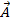Often in equations it is easiest to use only the magnitude of a vector. The magnitude of a vector can be identified by vertical lines on either side of the letter, or by the letter with the arrow removed. The magnitude of vectoris,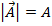Displacement
The term "distance" is used in physics to mean a scalar measurement, such as "3 meters". The term "displacement" is used to mean a vector quantity. Therefore, displacement has both a distance and a direction. When an object moves along a straight line, its starting position can be defined as the origin, O. The variable x can be assigned to mean any position along that line. The displacement is a vector that points from the origin to the position x. So, the displacement is the vector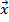.

To represent two or more positions along the straight line, the variables can be given numbers in subscript, for example, x1 and x2. If an object moves from position x1 to position x2, the change in the object's position is written as,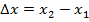The Greek uppercase letter ∆ ("delta") means "the change in". This change in position is a distance. The SI unit of displacement and distance measurements is the meter (m).

Velocity
To study moving objects, we need to understand how the movement relates to time. The term "speed" is used in physics to mean a scalar measurement, while the term "velocity" is used to mean a vector quantity. Velocity is the rate of change of an object's displacement as it moves from one place to another. The SI unit of velocity is meters per second, m/s. The magnitude of the velocity is the speed. Imagine that an object is at position x1 at a certain time t1. Then, it moves in a straight line so that it arrives at position x2 at time t2. Using ∆ to mean "the change in", the distance traveled is,The change in time can be written in the same way,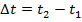The magnitude of the velocity, v, of an object is the distance traveled divided by the change in time,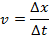The rate of change of ∆x divided by ∆t does not have to be constant. If an object speeds up or slows down, more or less distance is traveled in each unit of time. The velocity of the object at any particular time t is called the instantaneous velocity. However, between any two times the "average" velocity can be found. For ∆x = x2-x1 and ∆t = t2-t1, the average velocity is,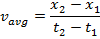There may be many different values of the velocity between the times t1 and t2. For the special case that the velocity is constant, then at any time between t1 and t2 the velocity's magnitude will be equal to vavg.

Acceleration
A change in velocity with respect to time is called acceleration. Acceleration is a vector quantity, with both magnitude and direction. Acceleration is the rate of change of an object's velocity. The SI unit of acceleration is meters per second squared (sometimes written as "per second per second"), m/s2. Imagine that at a time t1 an object is moving at a velocity with magnitude v1. Then, its velocity changes, so that at time t2 it is moving at a new velocity with magnitude v2. Using ∆ to mean "the change in", the change in the magnitude of the velocity can be written as,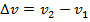The change in time can be written in the same way,The magnitude of the acceleration, a, of an object is the change in the magnitude of the object's velocity divided by the change in time,The rate of change of ∆v divided by ∆t does not have to be constant. The acceleration of the object at any particular time t is called the instantaneous acceleration. However, between any two times the "average" acceleration can be found. For ∆v = v2 - v1 and ∆t = t2 - t1, the magnitude of the average acceleration is,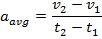There may be many different values of the acceleration between the times t1 and t2. In AP Physics, acceleration will almost always be taken to be constant. In this case, at any time between t1 and t2 the acceleration's magnitude will be equal to aavg.

 Related Links: Physics Quizzes AP Physics Notes Kinematic Formulas and Freefall